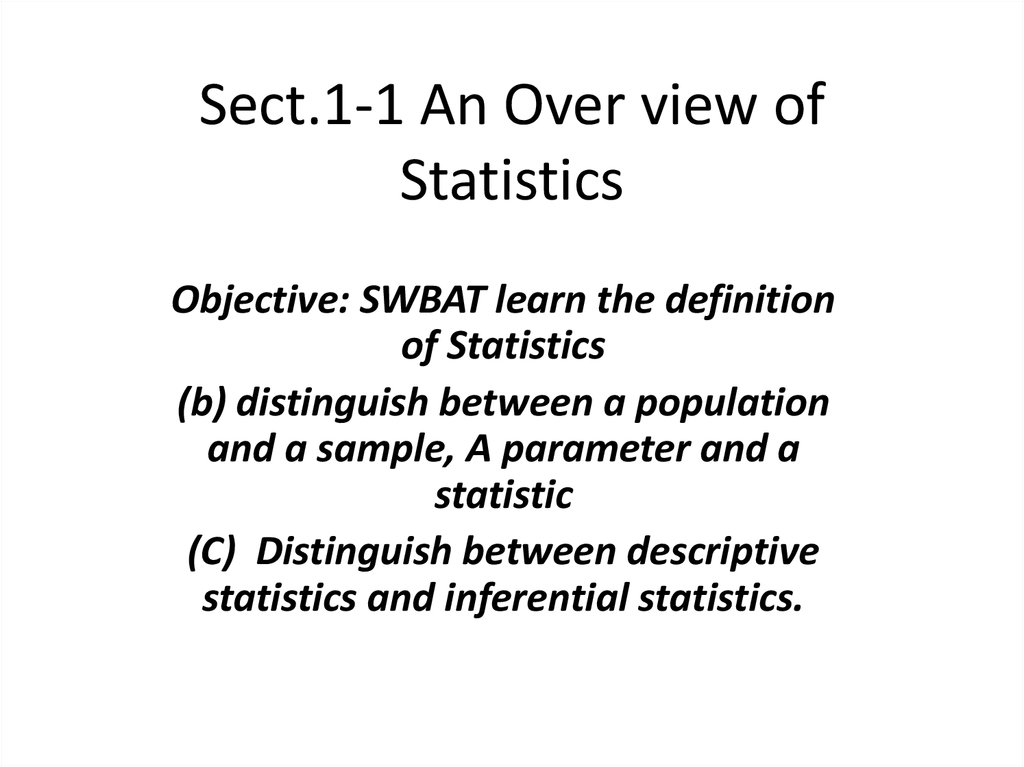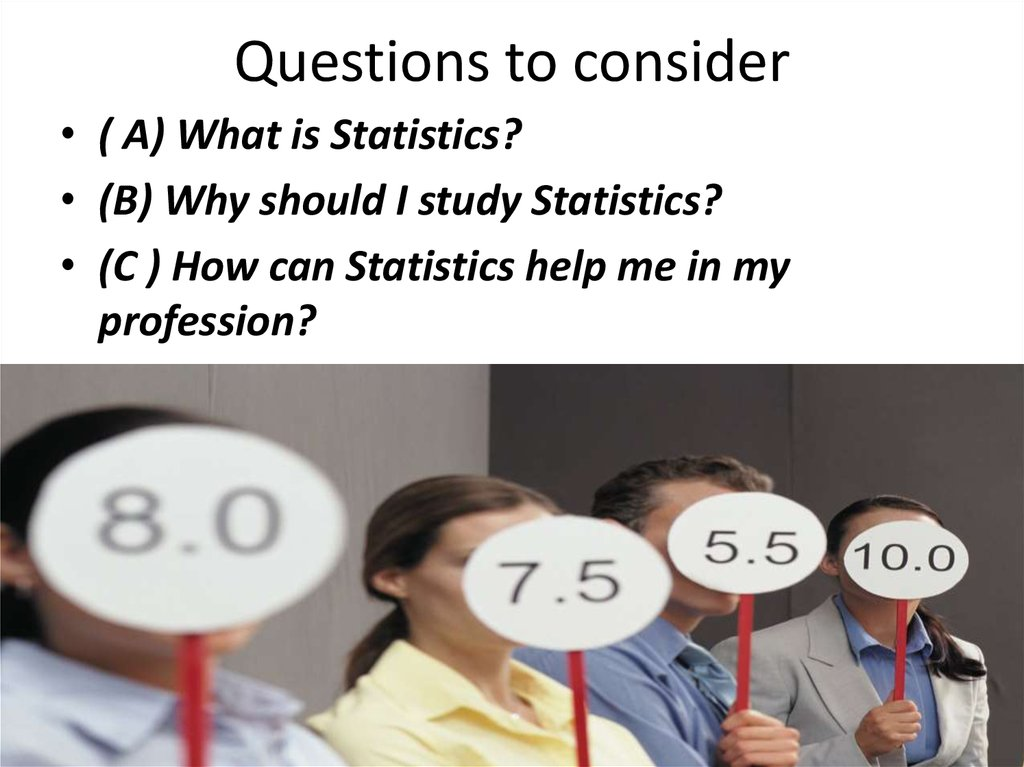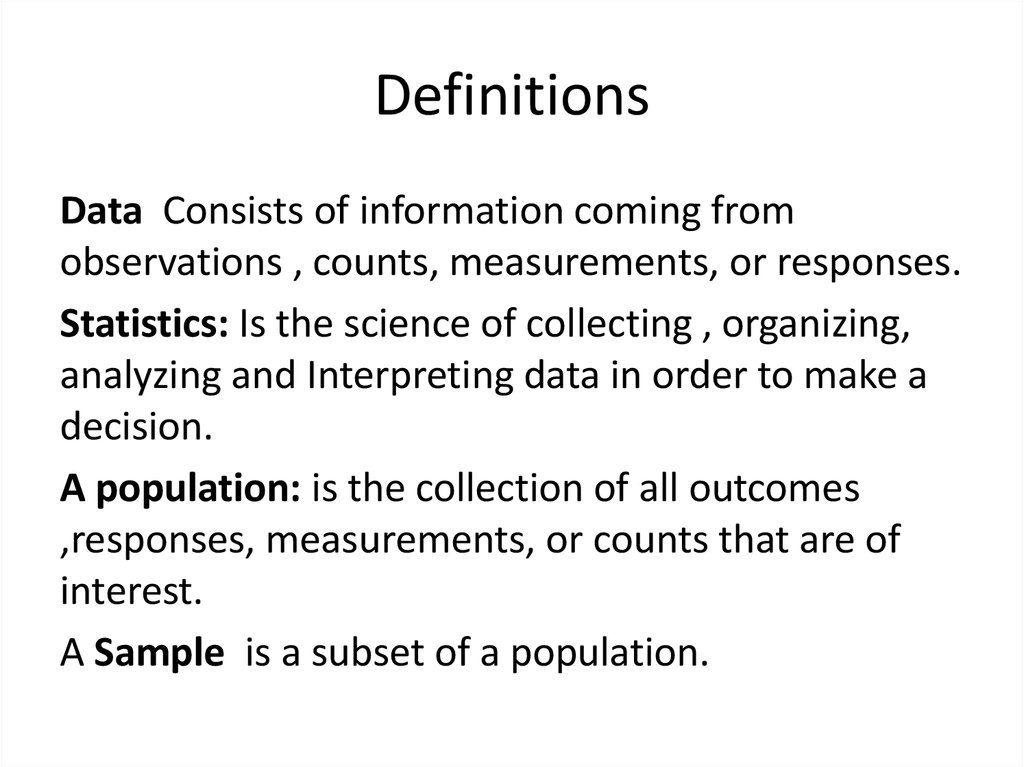# An over view of statistics

## 1. Sect.1-1 An Over view of Statistics

Objective: SWBAT learn the definition
of Statistics
(b) distinguish between a population
and a sample, A parameter and a
statistic
(C) Distinguish between descriptive
statistics and inferential statistics.

## 2. Questions to consider

• ( A) What is Statistics?
• (B) Why should I study Statistics?
• (C ) How can Statistics help me in my
profession?

## 3. Definitions

Data Consists of information coming from
observations , counts, measurements, or responses.
Statistics: Is the science of collecting , organizing,
analyzing and Interpreting data in order to make a
decision.
A population: is the collection of all outcomes
,responses, measurements, or counts that are of
interest.
A Sample is a subset of a population.

## 4. Examples

• Identifying Data Sets:
In a recent survey 3002 adults in the United
internet at least once a week. Six hundred of the
adults said Yes. Identify the population and the
sample. Describe the data set.
The population consists of the responses of all
the adults in the United States. And the Sample
consists of the 3002 adults in the United States.
The data set consists of 600 yes’s and 2402 no’s.

## 5. Venn Diagram

Responses of all adults in the United States
(population. )
the Survey
(Sample. )
600 yes’s
2402 No’s

## 6. Try this Yourself

The U.S. Department of Energy conducts
surveys of 800 gasoline stations to
determine the average price per gallon of
regular gasoline. On May 24,2001 the
average price was \$1.713 per gallon.
Identify the population and the Sample.

The population consists of the prices per gallon
of all stations in the United States.
The Sample consists of the prices per gallon of
regular gas at the 800 surveyed stations.
The data sets consists of the 800 prices.

## 8. Definitions

A parameter Is a numerical description of a
population characteristic.
A statistic is a numerical description of a sample
characteristic.

## 9. Examples

1.)A recent survey of a sample of MBA’s reported
that the average starting salary for an MBA is less
than \$65,000.
2.) Starting salaries for the 667 MBA graduates from
the University of Chicago Graduate School of
Business increased 8.5% from the previous year.
3.) In a random check of retail stores The Food and
Drug Administration found that 34% of the stores
were not storing fish at the proper temperature.

## 10. Solution

1.) because the numerical measure of \$65,000 is
based on a subset of the population it is a sample
statistic.
2.)Because the numerical measure of 8.5% is based
on all 667 graduates starting salaries it is a
population statistic.
3.) Because the numerical measure of34% is based
on a subset of the population it is a sample statistic.

## 11. Try This Yourself

In 2001 major league baseball teams spent a
total of \$1,968,088,814 on players salaries. Does
this numerical value represent a population
parameter or a sample statistic?
a.) It is Population
b.) It is a parameter.

## 12. Branches of Statistics

Definition
Descriptive Statistics: is the branch of statistics
that involves the organization ,summarization ,
and display of data.
Inferential Statistics: is the branch of statistics
that involves using a sample to draw conclusions
about a population. A basic tool in the study of
Inferential statistics is Probability.

## 13. Example

A large sample of men aged 48 were studied for
18 years. For unmarried men 60% to 70% were
alive at 65. For married men 90% were alive at
65. Which part represents descriptive Statistics?
What conclusions might be drawn from this
study using inferential Statistics?
Solution
Descriptive Statistics involves statements such as
For unmarried men 60% to 70% were alive at 65.
For married men 90% were alive at 65
A possible inference that might be drawn from this
study is that being married is associated with a

## 14. Example

In a sample of Wall Street analysts the percent
who incorrectly forecasted high-tech earnings in
a recent year was 44%.
Solution
The part of the study that represents the
descriptive branch of statistics involves the
statement “ The percent of Wall Street analysts
who incorrectly forecasted high-tech earnings in
a recent year was 44%”. A possible inference
drawn from the study is that the stock market is
difficult to forecast even for professionals.

## 16. Section 1.1

An Overview of
Statistics
After you see the slides for each section, do the Try It
Yourself problems in your text for that section to see if
you understood the material. Then, do the assigned
problems for that section.

## 17. Introduction to Statistics

As you view these slides be sure to have
paper, pencil, a calculator and your text
handy. Click to advance to the slide show.
17

## 18.

What Is Statistics?
Statistics is the science of
collecting, organizing,
analyzing, and interpreting data
in order to make decisions.
18

## 19. Important Terms

Population
The collection of all responses,
measurements, or counts that are of interest.
Sample
A portion or subset of the population.
19

## 20. Important Terms

Parameter
A number that describes a population characteristic.
Average gross income of all
people in the United States in
2002.
Statistic
A number that describes a sample characteristic.
2002 gross income of people
from a sample of three states.
20

## 21. Two Branches of Statistics

Descriptive Statistics
Involves organizing, summarizing, and displaying
data.
Inferential Statistics
Involves using sample data to draw conclusions